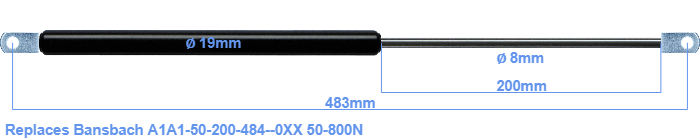# Replacement for Bansbach A1A1-50-200-484–0XX 50-800N

\$36.31

Replacement gas spring for the Bansbach A1A1-50-200-484--0XX 50-800 Newton. The eye has a thickness of 10mm and a hole diameter of 8.1mm. Brand: Hahn.
 Force Choose an option50 Newton60 Newton80 Newton100 Newton120 Newton140 Newton150 Newton160 Newton180 Newton200 Newton220 Newton240 Newton250 Newton260 Newton280 Newton300 Newton320 Newton340 Newton350 Newton360 Newton380 Newton400 Newton420 Newton440 Newton450 Newton460 Newton480 Newton500 Newton520 Newton540 Newton550 Newton560 Newton580 Newton600 Newton620 Newton640 Newton650 Newton660 Newton680 Newton700 Newton720 Newton740 Newton750 Newton760 Newton780 Newton800 NewtonClearThe bore of the house of this replacement gas spring is 19 mm. The diameter of the rod is 8 millimeter. The stroke is 200 millimeter long. The total length of this replacement is exactly 483 mm. This is the distance between the centers of the mounting parts. Without the mounting parts this gas spring is 445 mm long (thread to thread). This replacement gas spring (A1A1-50-200-484--0XX) has a force of 50-800 Newton (N). This is not an official Bansbach gas spring, but a so called replacement. HAHN Gasfedern, a Stabilus subsidiary, is responsible for the production of this replacement gas spring. The gas spring has the brand Stabilus Industry Line. Nevertheless, the force and dimensions equal.